# 7 1 class Figure double x double y

• Slides: 49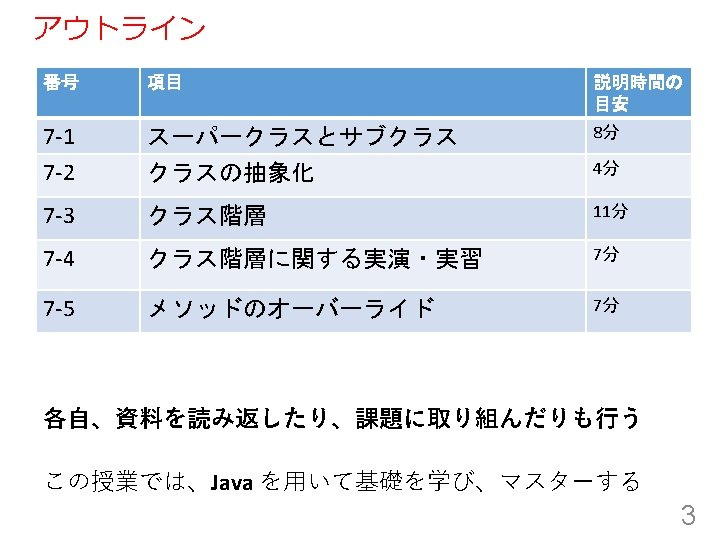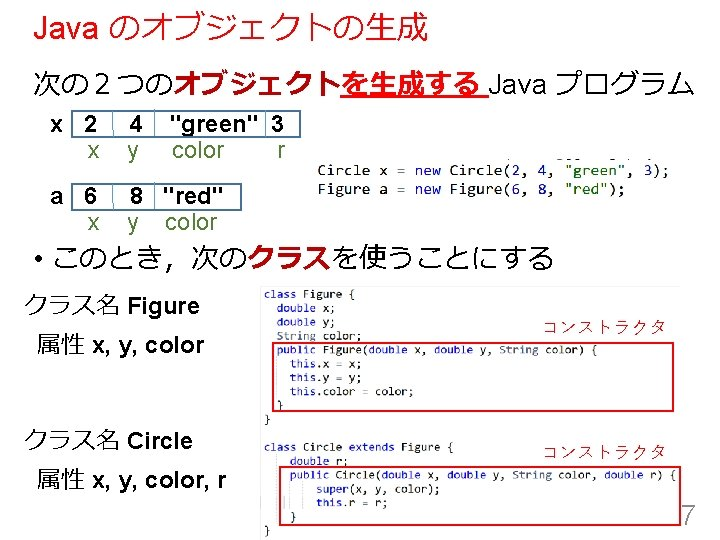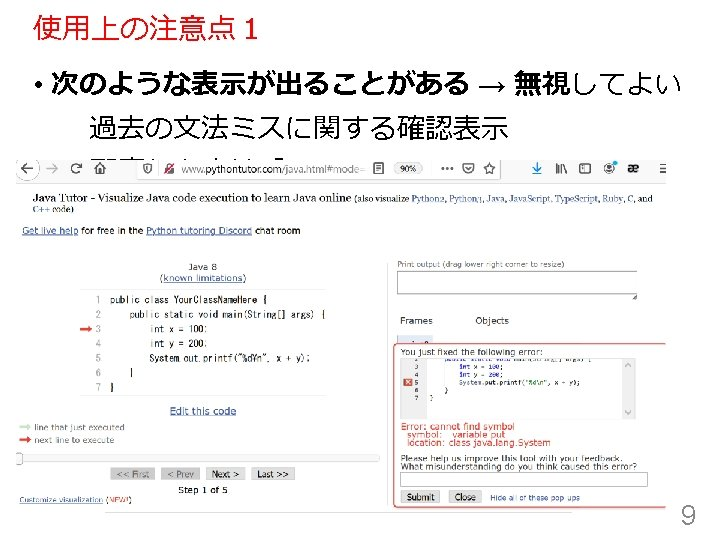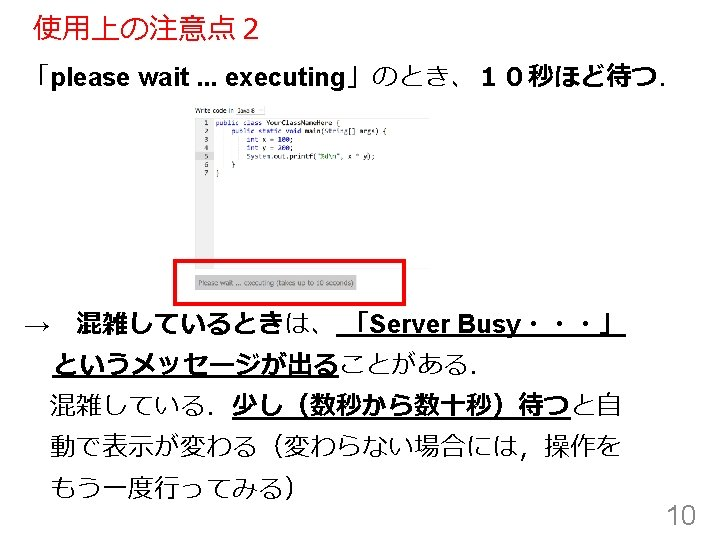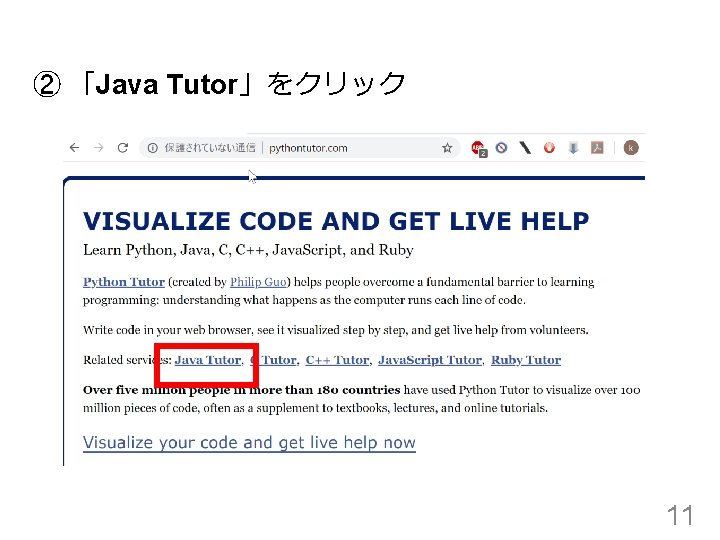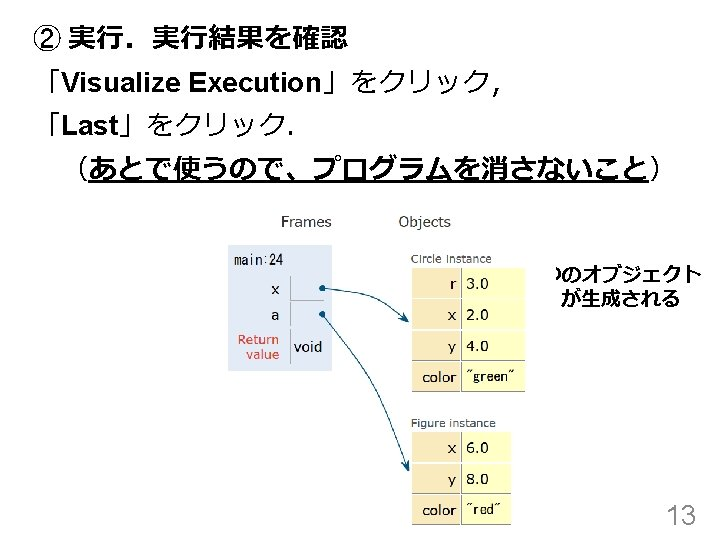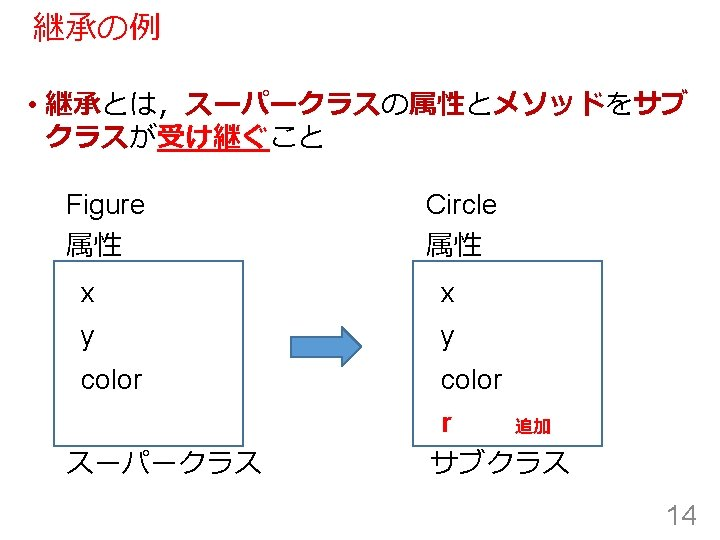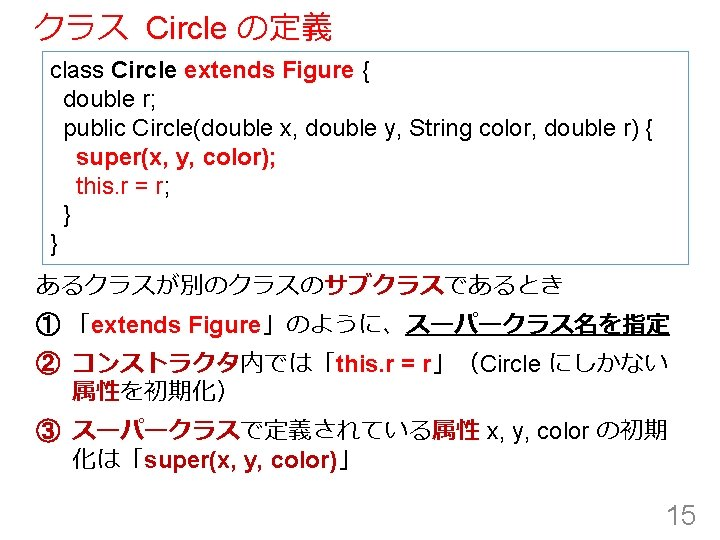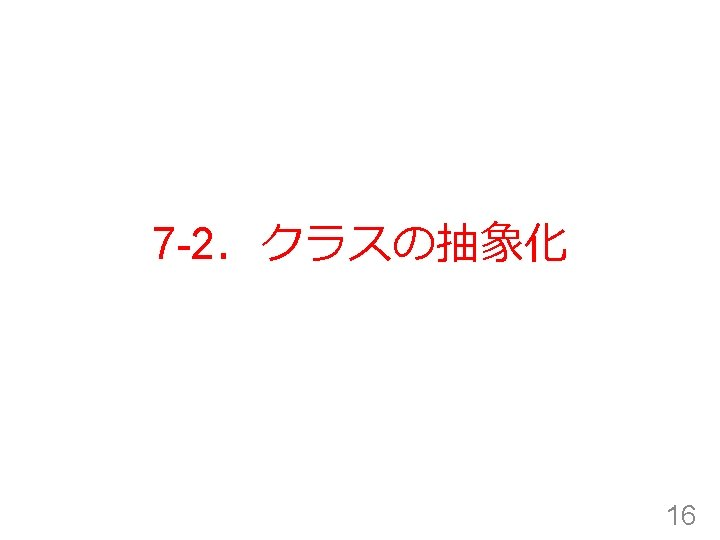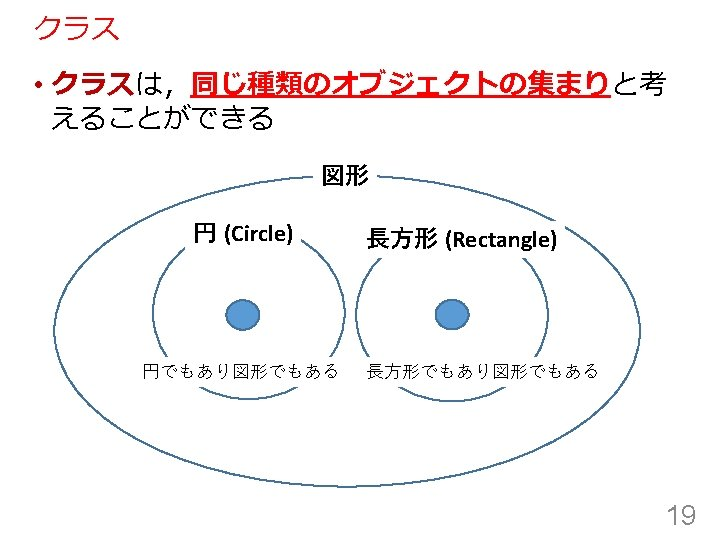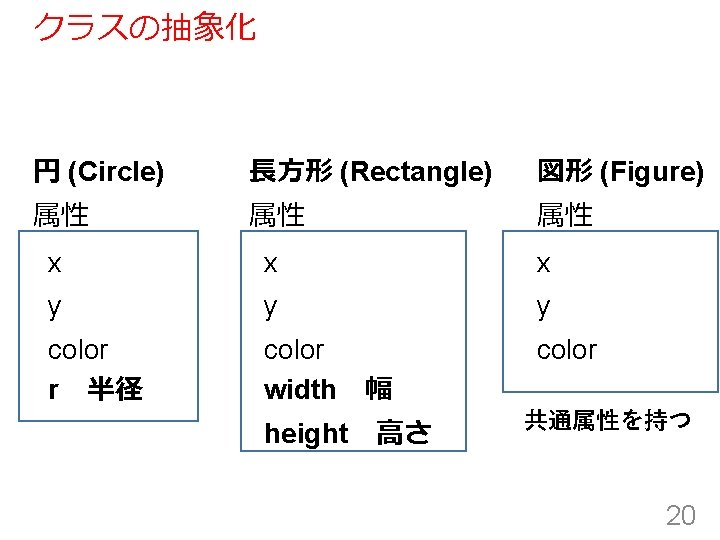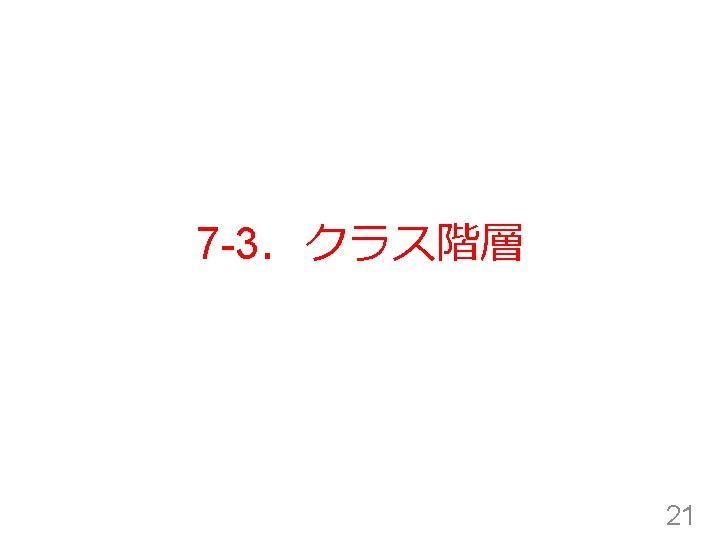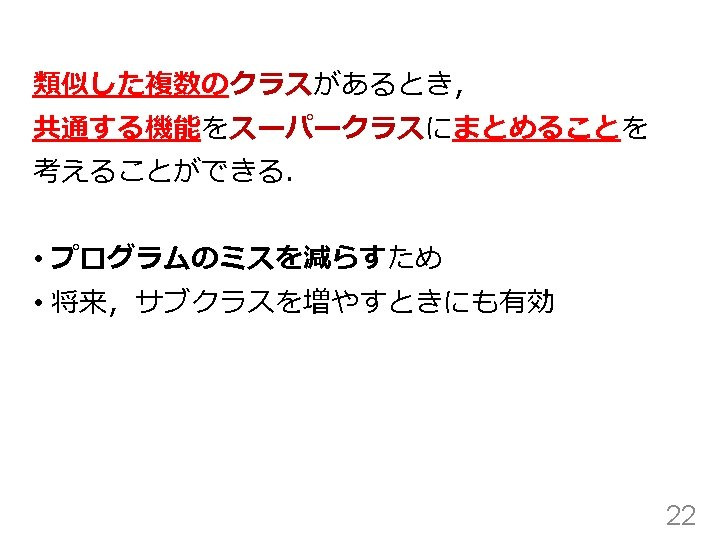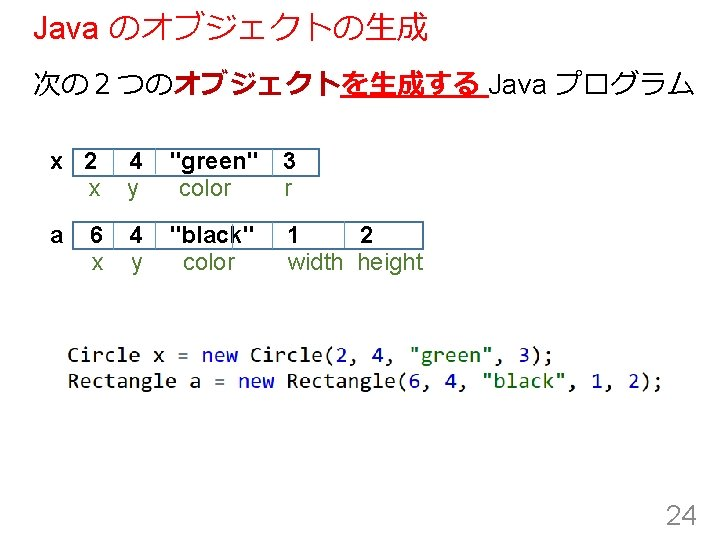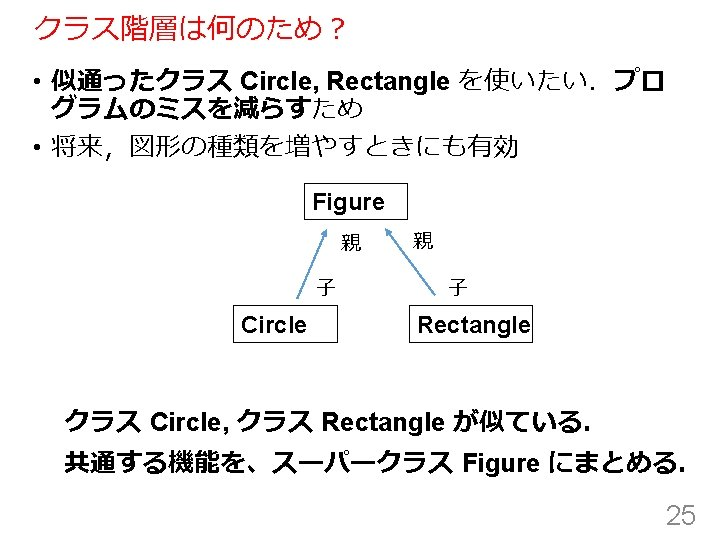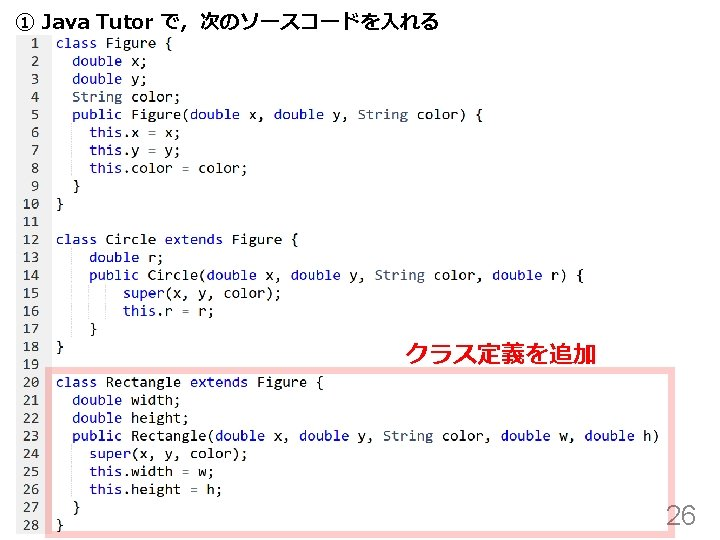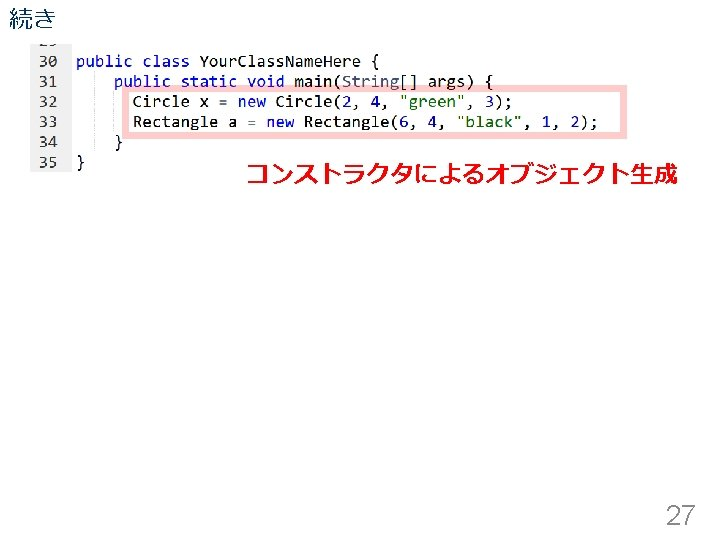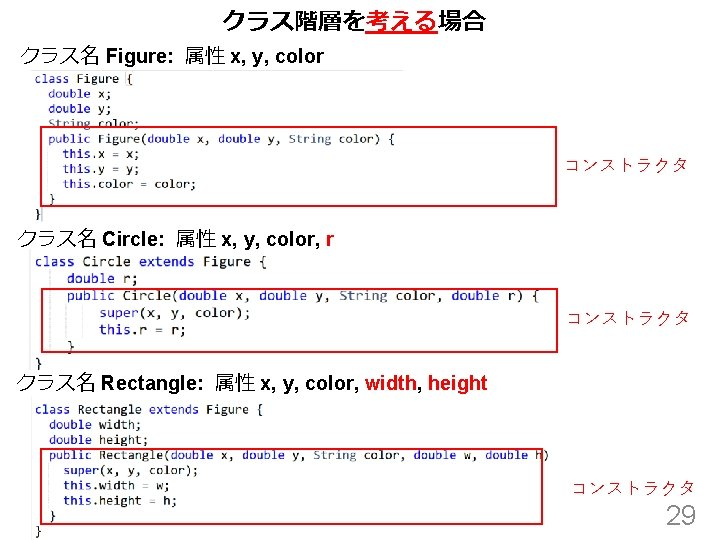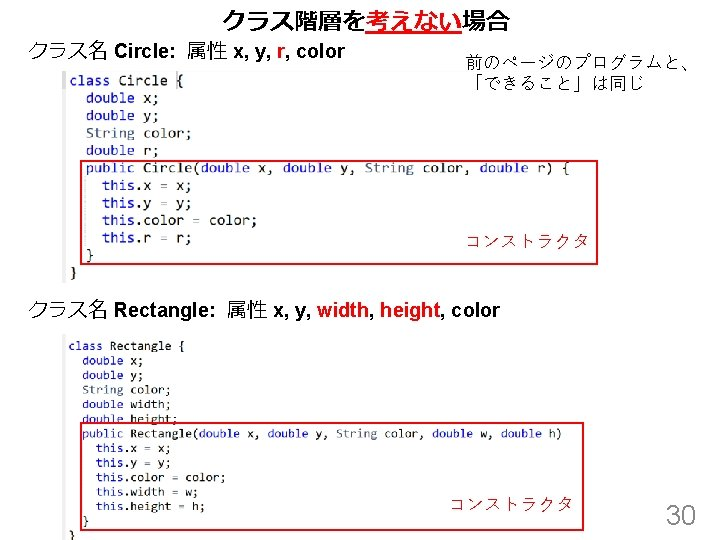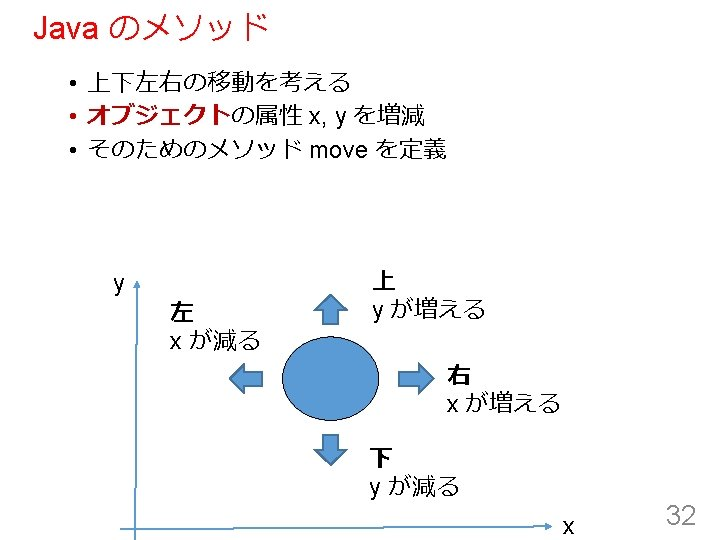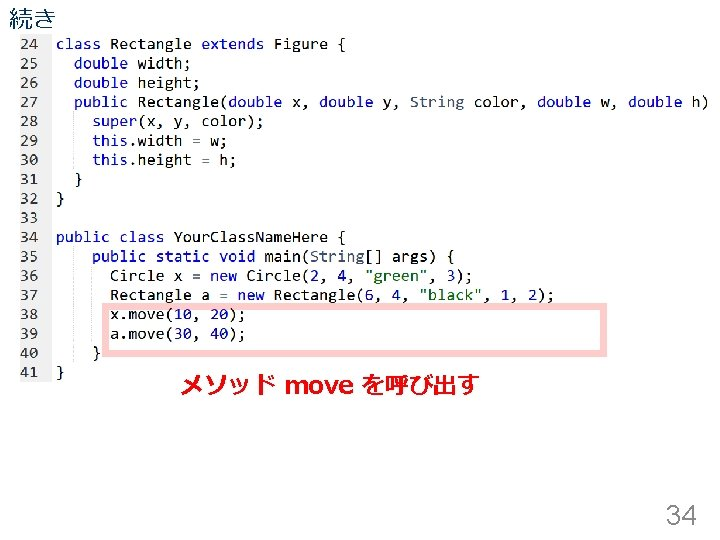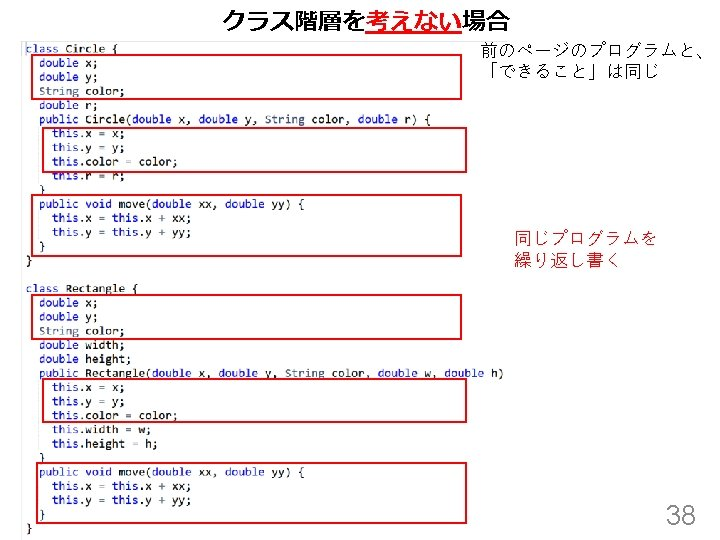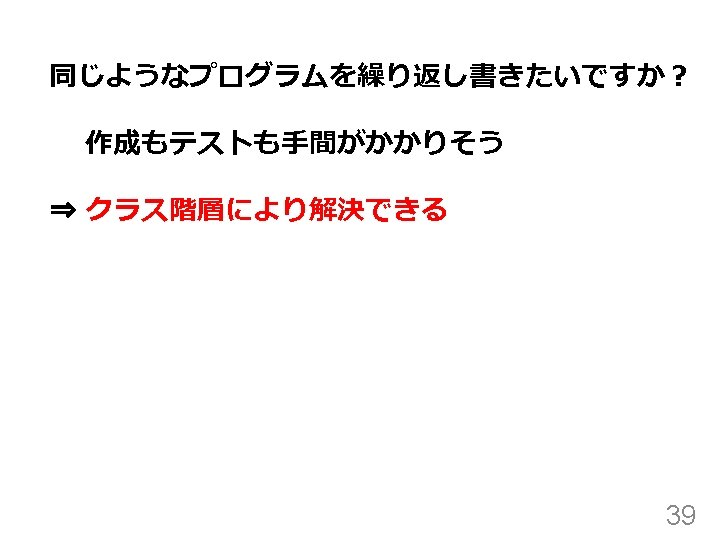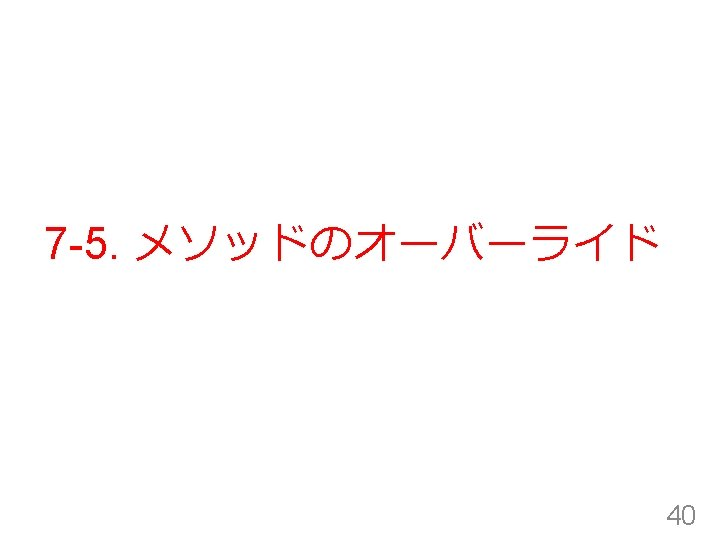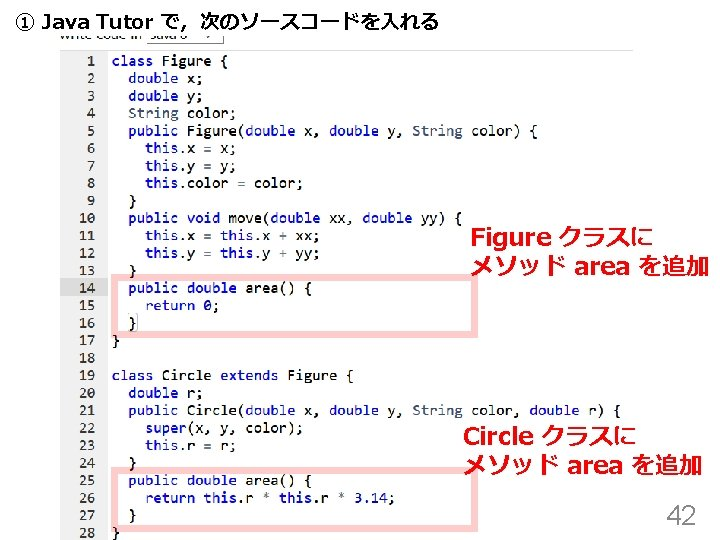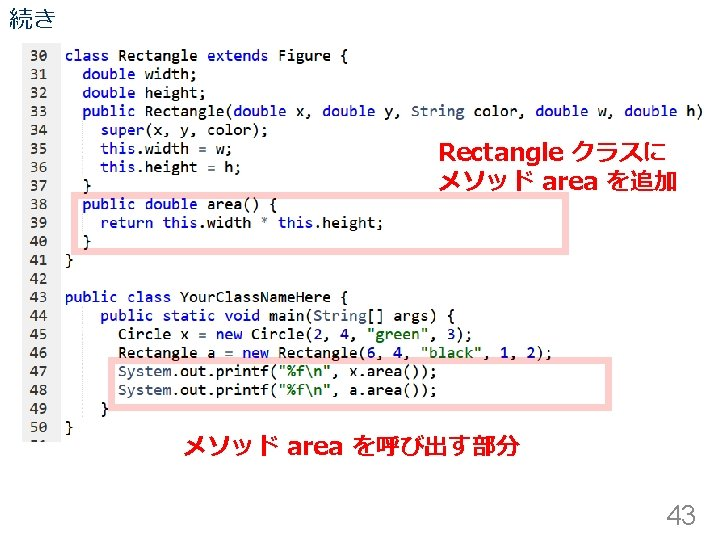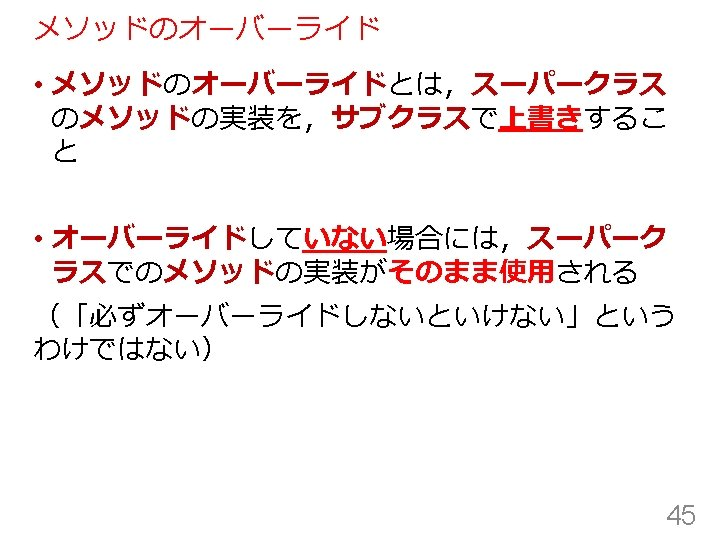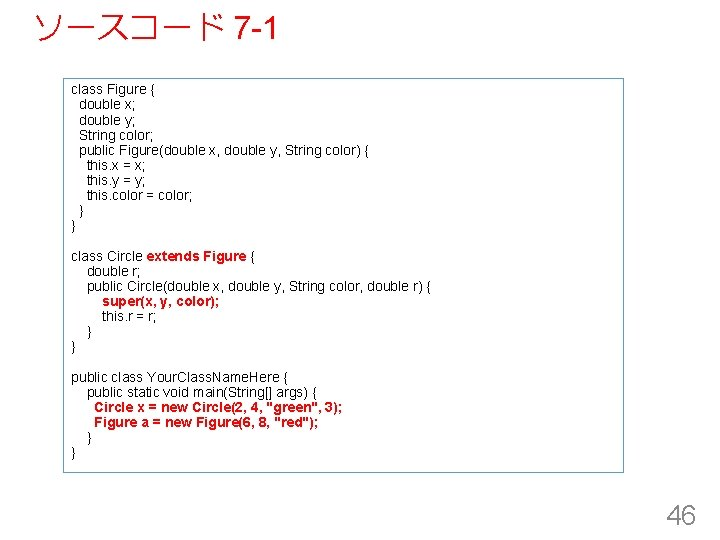ソースコード 7 -1 class Figure { double x; double y; String color; public Figure(double x, double y, String color) { this. x = x; this. y = y; this. color = color; } } class Circle extends Figure { double r; public Circle(double x, double y, String color, double r) { super(x, y, color); this. r = r; } } public class Your. Class. Name. Here { public static void main(String[] args) { Circle x = new Circle(2, 4, "green", 3); Figure a = new Figure(6, 8, "red"); } } 46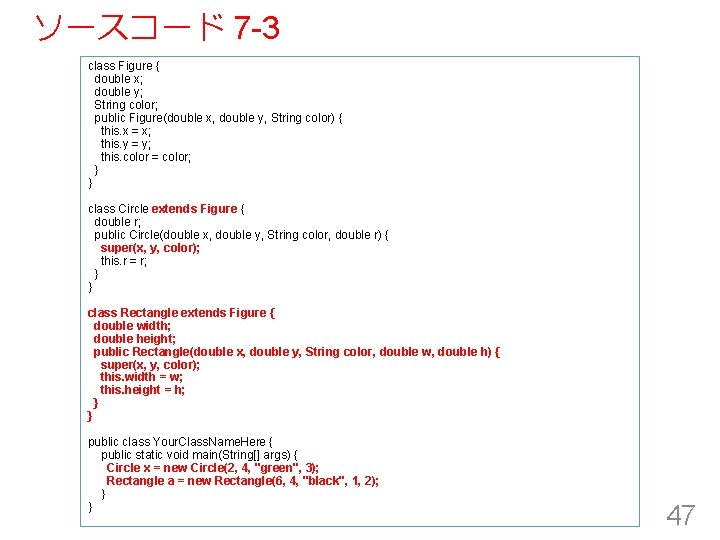ソースコード 7 -3 class Figure { double x; double y; String color; public Figure(double x, double y, String color) { this. x = x; this. y = y; this. color = color; } } class Circle extends Figure { double r; public Circle(double x, double y, String color, double r) { super(x, y, color); this. r = r; } } class Rectangle extends Figure { double width; double height; public Rectangle(double x, double y, String color, double w, double h) { super(x, y, color); this. width = w; this. height = h; } } public class Your. Class. Name. Here { public static void main(String[] args) { Circle x = new Circle(2, 4, "green", 3); Rectangle a = new Rectangle(6, 4, "black", 1, 2); } } 47ソースコード 7 -4 class Figure { double x; double y; String color; public Figure(double x, double y, String color) { this. x = x; this. y = y; this. color = color; } public void move(double xx, double yy) { this. x = this. x + xx; this. y = this. y + yy; } } class Circle extends Figure { double r; public Circle(double x, double y, String color, double r) { super(x, y, color); this. r = r; } } class Rectangle extends Figure { double width; double height; public Rectangle(double x, double y, String color, double w, double h) { super(x, y, color); this. width = w; this. height = h; } } public class Your. Class. Name. Here { public static void main(String[] args) { Circle x = new Circle(2, 4, "green", 3); Rectangle a = new Rectangle(6, 4, "black", 1, 2); x. move(10, 20); a. move(30, 40); } } 48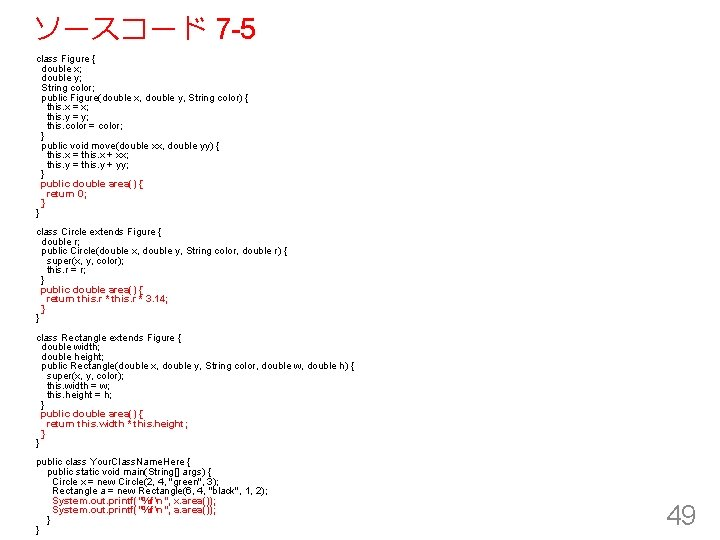ソースコード 7 -5 class Figure { double x; double y; String color; public Figure(double x, double y, String color) { this. x = x; this. y = y; this. color = color; } public void move(double xx, double yy) { this. x = this. x + xx; this. y = this. y + yy; } public double area() { return 0; } } class Circle extends Figure { double r; public Circle(double x, double y, String color, double r) { super(x, y, color); this. r = r; } public double area() { return this. r * 3. 14; } } class Rectangle extends Figure { double width; double height; public Rectangle(double x, double y, String color, double w, double h) { super(x, y, color); this. width = w; this. height = h; } public double area() { return this. width * this. height; } } public class Your. Class. Name. Here { public static void main(String[] args) { Circle x = new Circle(2, 4, "green", 3); Rectangle a = new Rectangle(6, 4, "black", 1, 2); System. out. printf("%fn", x. area()); System. out. printf("%fn", a. area()); } } 49